# numpy.exp¶

numpy.exp(x[, out])

Calculate the exponential of all elements in the input array.

Parameters : x : array_like Input values. out : ndarray Output array, element-wise exponential of x.

expm1
Calculate exp(x) - 1 for all elements in the array.
exp2
Calculate 2**x for all elements in the array.

Notes

The irrational number e is also known as Euler’s number. It is approximately 2.718281, and is the base of the natural logarithm, ln (this means that, if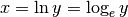, then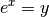. For real input, exp(x) is always positive.

For complex arguments, x = a + ib, we can write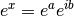. The first term,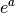, is already known (it is the real argument, described above). The second term,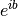, is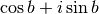, a function with magnitude 1 and a periodic phase.

References

 [R18] Wikipedia, “Exponential function”, http://en.wikipedia.org/wiki/Exponential_function
 [R19] M. Abramovitz and I. A. Stegun, “Handbook of Mathematical Functions with Formulas, Graphs, and Mathematical Tables,” Dover, 1964, p. 69, http://www.math.sfu.ca/~cbm/aands/page_69.htm

Examples

Plot the magnitude and phase of exp(x) in the complex plane:

>>> import matplotlib.pyplot as plt

>>> x = np.linspace(-2*np.pi, 2*np.pi, 100)
>>> xx = x + 1j * x[:, np.newaxis] # a + ib over complex plane
>>> out = np.exp(xx)

>>> plt.subplot(121)
>>> plt.imshow(np.abs(out),
...            extent=[-2*np.pi, 2*np.pi, -2*np.pi, 2*np.pi])
>>> plt.title('Magnitude of exp(x)')

>>> plt.subplot(122)
>>> plt.imshow(np.angle(out),
...            extent=[-2*np.pi, 2*np.pi, -2*np.pi, 2*np.pi])
>>> plt.title('Phase (angle) of exp(x)')
>>> plt.show()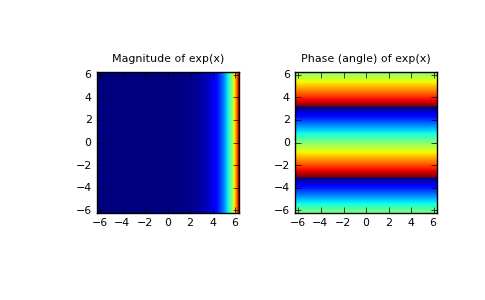numpy.trapz

numpy.expm1